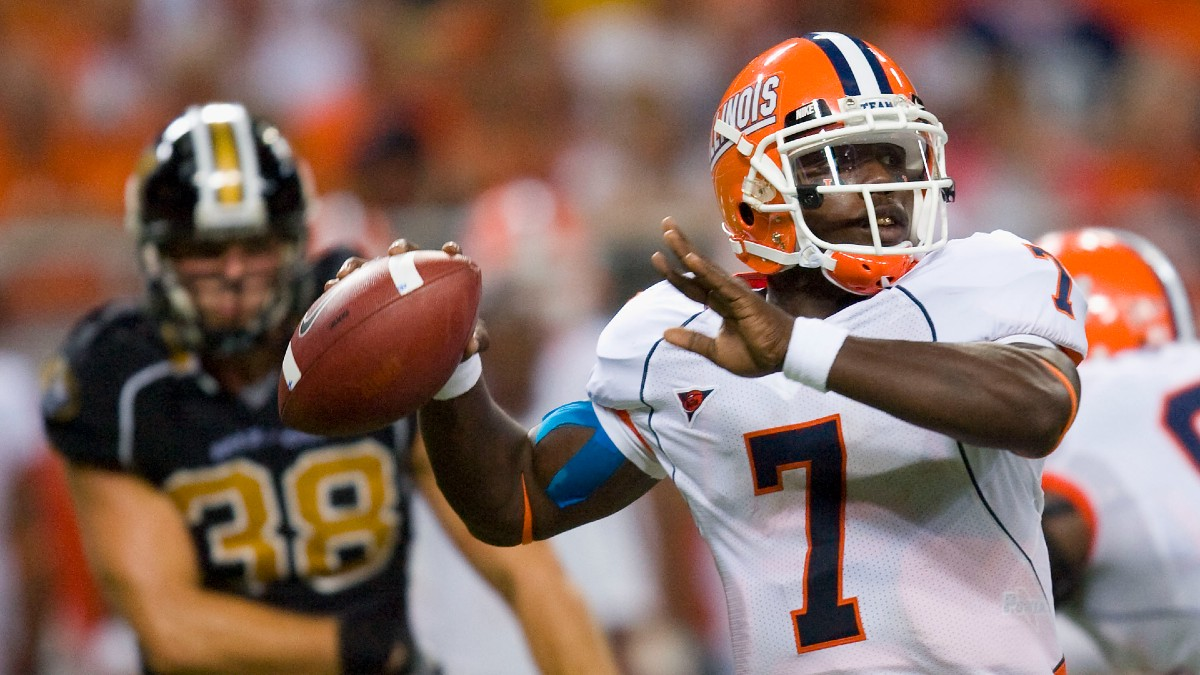# How to Remove Juice/Vig from Sports Betting OddsCredit:

Getty Images. Pictured: Illinois quarterback Juice Williams

The probabilities of two outcomes in sports betting never add up to 100%. That’s because sportsbooks take their cut — called the juice or vig.

But to truly see how a sportsbook views an event, you need to remove that vig.

Here’s how to do it.

## How Implied Probability Works

Before we can remove the vig, we need to figure out what it is.

You’ll need to convert American odds to implied probability, then add those two probabilities together. The percentage that exceeds 100% is the vig.

Let’s use a moneyline in which the Packers are -200 and the Vikings are +170 as an example.

The formulas to convert American odds to implied probabilities will be slightly different if you’re converting a negative moneyline (favorite) or positive moneyline (underdog).

Favorite: Odds / (Odds + 100) *100

Packers: 200 / (200 + 100) *100 = 66.667%

That implies the Packers have about a 66.7% chance to win this game.

Underdog: 100 / (Odds + 100) *100

Vikings: 100 / (Odds + 100) *100 = 37.04%

That implies the Vikings have about a 37% chance to win this game.

Those two probabilities add up to 103.7%.

You can use this formula and it will convert either a favorite or underdog:

=IFERROR(IF(B2>0,1/(B2/100+1),1/(1+100/ABS(B2))))

## How to Remove the Juice

Removing the juice is not as simple as dividing 3.7% by two and subtracting from each side, but it’s fairly straightforward.

Here’s the formula:

Team A Implied Probability / (Team A IP + Team B IP)

Packers: 45.45% / (45.45% + 58.33%) = 44%

Vikings: 58.33% / (58.33% + 45.45%) = 56%

That gets you to 100%.

Here’s a quick video tutorial on how to remove juice in Excel.

## Why Remove the Juice?

It’s important to remove the juice on each implied probability so you can see what true odds are for each game. That way, you’re getting a more accurate picture of what the sportsbook and betting market really thinks of each team.

If you’re projecting games on your own using some sort of formula or model, you’ll want to compare to the true price to calculate your edge.

If you’d like to learn more about betting on football, here is a list of recommended resources to check out: